International
Tables for
Crystallography
Volume B
Reciprocal space
Edited by U. Shmueli

International Tables for Crystallography (2006). Vol. B, ch. 5.1, p. 539   | 1 | 2 |

Section 5.1.3.4. Deviation parameter

A. Authiera*

aLaboratoire de Minéralogie-Cristallographie, Université P. et M. Curie, 4 Place Jussieu, F-75252 Paris CEDEX 05, France
Correspondence e-mail: authier@lmcp.jussieu.fr

5.1.3.4. Deviation parameter

| top | pdf |

The solutions of dynamical theory are best described by introducing a reduced parameter called the deviation parameter,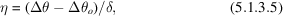where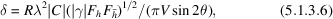whose real part is equal to the half width of the rocking curve (Sections 5.1.6and 5.1.7). The width 2δ of the rocking curve is sometimes called the Darwin width.

The definition (5.1.3.5)of the deviation parameter is independent of the geometrical situation (reflection or transmission case); this is not followed by some authors. The present convention has the advantage of being quite general.

In an absorbing crystal,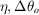and δ are complex, and it is the real part,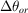, of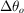which has the geometrical interpretation given in Section 5.1.3.3. One obtains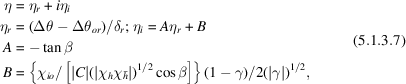where β is the phase angle of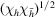[or that of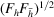].